Author: Oscar Cronquist Article last updated on November 22, 2021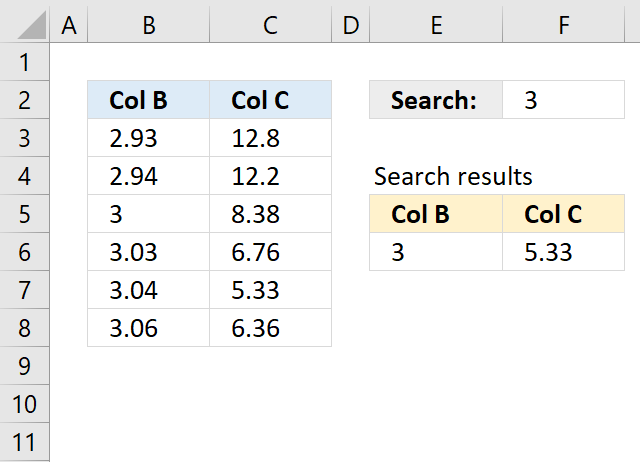This article demonstrates how to extract the smallest number larger than a condition and the largest number smaller than a condition.

## 1. Get the smallest number larger than a given number - Excel 2016

Question:

Here is the problem:
i have a data table with 2 columns:
A B
2.93 12.8
2.94 12.2
3 8.38
3.03 6.76
3.04 5.33
3.06 6.36
Lets say i have a cell with number 3. I need to find a number in column A that has a number >= than 3, but also has the smallest number in column B.
(with my cell = 3 it would be 3.04 from A and 5.33 from B)
Simple vlookup gives me first >= number, but in most cases in column B is not the smallest number.The formula extracts the smallest number larger than a given number displayed in cell F2.

Formula in E6:

=MINIFS(B3:B8,B3:B8,">"&F2)

The MINIFS function calculates the smallest value based on a given set of criteria.

MINIFS(min_rangecriteria_range1criteria1, [criteria_range2criteria2], ...)

min_range - Required. A reference to the numbers.
criteria_range1 -  A reference to cells to evaluate based on the criteria.
criteria1 - Criteria in the form of a number, expression, or text.

Formula in cell F6:

=MINIFS(C3:C8,C3:C8,">"&F2)

MINIFS function was introduced in Excel 2016, you can use the following formulas if you don't have access to the function.

### 1.1 Get the smallest number larger than a given number - Previous Excel versionsArray formula in E6 for previous Excel versions:

=MIN(IF(B3:B8>F2,B3:B8))

Array formula in F6 for previous Excel versions:

=MIN(IF(C3:C8>F2,C3:C8))

### 1.2 Explaining formula for previous versions in cell E6

MIN(IF(B3:B8>F2,B3:B8))

#### Step 1 - Construct logical expression

The logical expression is used in the IF function in order to return a given value. The > is a larger than sign.

B3:B8>F2

becomes

{2.93;2.94;3;3.03;3.04;3.06}>3

and returns

{FALSE; FALSE; FALSE; TRUE; TRUE; TRUE}

#### Step 2 - Extract values in array

IF(B3:B8>F2,B3:B8)

becomes

IF(B3:B8>F2,B3:B8)

becomes

IF({FALSE; FALSE; FALSE; TRUE; TRUE; TRUE},{2.93;2.94;3;3.03;3.04;3.06})

and returns

{FALSE; FALSE; FALSE; 3.03; 3.04; 3.06}

#### Step 3 - Find smallest value

The MIN function ignores text, blank and boolean values.

MIN(IF(B3:B8>F2,B3:B8))

becomes

MIN({FALSE; FALSE; FALSE; 3.03; 3.04; 3.06})

and returns 3.03 in cell E6.

## 2. Get the largest number smaller than a given number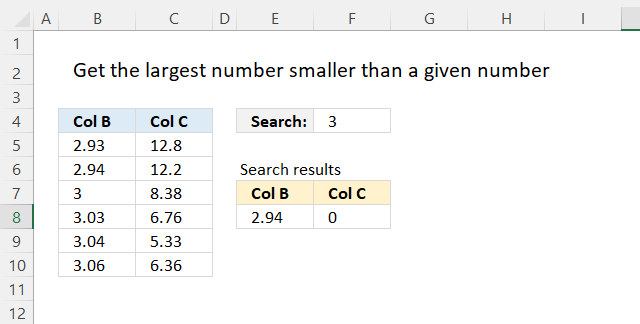Formula in cell E8:

=MAXIFS(B3:B8,B3:B8,"<"&F2)

Formula in cell F8:

=MAXIFS(C3:C8,C3:C8,"<"&F2)

The MAXIFS function returns the largest number based on a given set of criteria.

MAXIFS(max_rangecriteria_range1criteria1, [criteria_range2criteria2], ...)

min_range - Required. A reference to the numbers.
criteria_range1  A reference to cells to evaluate based on the criteria.
criteria1 - Criteria in the form of a number, expression, or text.

### 2.1 Get the largest number smaller than a given number - Previous Excel versionsArray formula in E6 for previous Excel versions:

=MIN(IF(B3:B8>F2,B3:B8))

Array formula in F6 for previous Excel versions:

=MIN(IF(C3:C8>F2,C3:C8))

## 3. Get the smallest number larger than a given number and a condition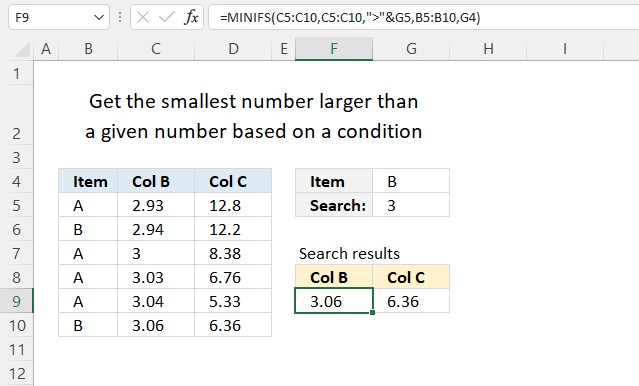Formula in E6:

=MINIFS(B3:B8,B3:B8,">"&F2, B5:B10, G4)

The MINIFS function calculates the smallest value based on a given set of criteria.

Formula in cell F6:

=MINIFS(C3:C8,C3:C8,">"&F2, B5:B10, G4)

MINIFS(min_rangecriteria_range1criteria1, [criteria_range2criteria2], ...)

min_range - Required. A reference to the numbers.
criteria_range1 -  A reference to cells to evaluate based on the criteria.
criteria1 - Criteria in the form of a number, expression, or text.

MINIFS function was introduced in Excel 2016, you can use the following formulas if you don't have access to the function.

## 4. Get the smallest number larger than a given number in a date range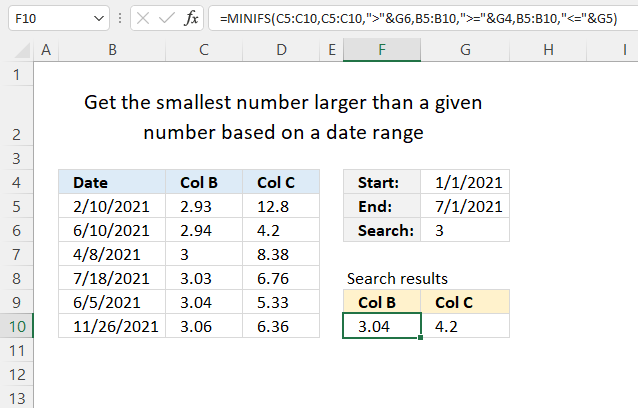Formula in cell F10:

=MINIFS(C5:C10,C5:C10,">"&G6,B5:B10,">="&G4,B5:B10,"<="&G5)

### 4.1 Explaining formula in cell F10

MINIFS(min_rangecriteria_range1criteria1, [criteria_range2criteria2], ...)

#### Step 1 - First condition

The first criteria_range1 is C5:C10, the first criteria1 is ">"&G6.

The ampersand character concatenates the larger than character and the condition specified in cell G6.

C5:C10,">"&G6

The first criteria pair checks if the values in C5:C10 are larger than the value in cell G6.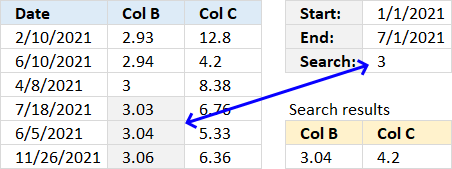The image above highlights which cells contain a number larger than the condition.

#### Step 2 - Second condition

The second criteria_range2 is B5:B10, the second criteria2 is ">="&G4.

The ampersand character concatenates the larger than character, the equal sign, and the condition specified in cell G4.

B5:B10,">="&G4

The second criteria pair checks if the dates in B5:B10 are later or equal to the date in cell G4.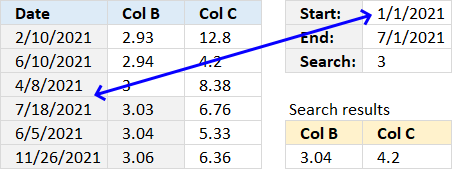The image above shows that all dates are equal or later than the start range date specified in cell G4.

#### Step 3 - Third condition

The third criteria_range2 is again B5:B10, the third criteria2 is "<="&G5.

The ampersand character concatenates the less than character, the equal sign, and the condition specified in cell G4.

B5:B10,"<="&G5

The second criteria pair checks if the dates in B5:B10 are later or equal to the date in cell G4.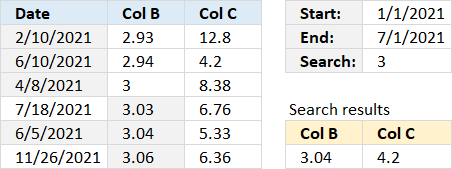The image above highlights dates equal to or earlier than the start range date specified in cell G4.

#### Step 4 - Evaluate MINIFS function

The MINIFS function calculates the smallest value based on a given set of criteria.

MINIFS(min_rangecriteria_range1criteria1, [criteria_range2criteria2], ...)

MINIFS(C5:C10,C5:C10,">"&G6,B5:B10,">="&G4,B5:B10,"<="&G5)

returns 3.04 in cell F10. When all conditions are met value 3.04 is returned.

#### Formula in cell G10

MINIFS(D5:D10,D5:D10,">"&G6,B5:B10,">="&G4,B5:B10,"<="&G5)

returns 4.2. Values 4.2 and 5.33 meet all conditions, however, 4.2 is the smallest.